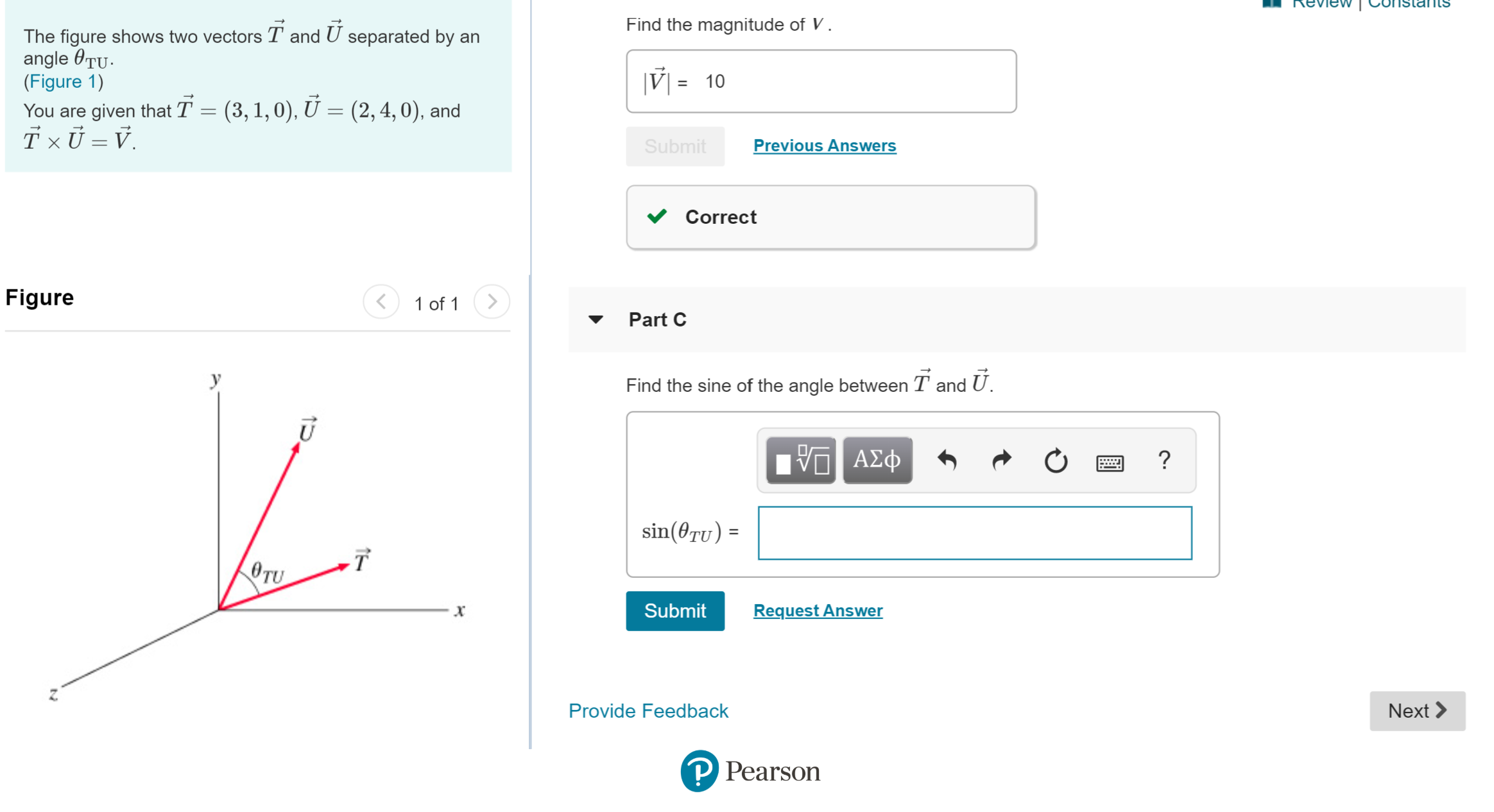# Find the magnitude of VThe figure shows two vectors T and U separated by anangle TU(Figure 1)10You are given that T = (3,1,0), U = (2,4,0), andSubmitPrevious AnswersCorrectFigure1 of 1Part CуFind the sine of the angle between T and U.ΑΣφ?sin(0TU) =TOTURequest AnswerSubmitXProvide FeedbackNextP Pearson

Question
6 viewshelp_outlineImage TranscriptioncloseFind the magnitude of V The figure shows two vectors T and U separated by an angle TU (Figure 1) 10 You are given that T = (3,1,0), U = (2,4,0), and Submit Previous Answers Correct Figure 1 of 1 Part C у Find the sine of the angle between T and U. ΑΣφ ? sin(0TU) = T OTU Request Answer Submit X Provide Feedback Next P Pearson fullscreen
check_circle

Step 1

Given

Step 2

Use the cross-pro...

### Want to see the full answer?

See Solution

#### Want to see this answer and more?

Solutions are written by subject experts who are available 24/7. Questions are typically answered within 1 hour.*

See Solution
*Response times may vary by subject and question.
Tagged in

### Vectors and Scalars